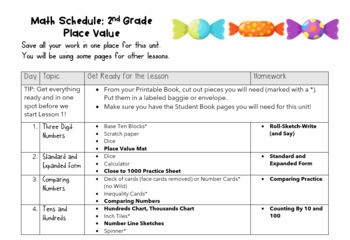# 2nd Grade Place Value--REMOTE edition2nd - 3rd
Subjects
Standards
Resource Type
Formats Included
•Google Drive™ folder### Description

NEW to this Unit! If you loved our beginning of the year review, this unit provides the next set of lessons, AND we have also included PDFs of all homework, exit tickets, and the choice board in a format that is SeeSaw-friendly!

This two-week lesson plan pack comes with printables, online activities, and digital versions of practice that will help your second graders tackle place value and addition and subtraction of multidigit numbers. It also includes all your lessons in a ready-to-go animated Google Slideshow, so you can demonstrate games and strategies in a remote classroom environment.

This unit was designed to cover all the second grade standards for Numbers and Operations in Base Ten:

Understand place value.

• Understand that the three digits of a three-digit number represent amounts of hundreds, tens, and ones. Understand the following as special cases:
• 100 can be thought of as a bundle of ten tens.
• The numbers 100, 200, 300, 400, 500, 600, 700, 800, 900 refer to one, two, three, four, five, six, seven, eight, or nine hundreds (and 0 tens and 0 ones).
• Count within 1000; skip-count by 5s, 10s, and 100s.
• Read and write numbers to 1000 using base-ten numerals, number names, and expanded form.
• Compare two three-digit numbers based on meanings of the hundreds, tens, and ones digits, using >, =, and < symbols to record the results of comparisons.

Use place value understanding and properties of operations to add and subtract.

• Fluently add and subtract within 100 using strategies based on place value, properties of operations, and/or the relationship between addition and subtraction.
• Add up to four two-digit numbers using strategies based on place value and properties of operations.
• Add and subtract within 1000, using concrete models or drawings and strategies based on place value; relate the strategy to a written method. Understand that in adding or subtracting three-digit numbers, one adds or subtracts hundreds and hundreds, tens and tens, ones and ones; and sometimes it is necessary to compose or decompose tens or hundreds.
• Mentally add 10 or 100 to a given number 100-900, and mentally subtract 10 or 100 from a given number 100-900.
• Explain why addition and subtraction strategies work, using place value and the properties of operations.
Total Pages
Included
Teaching Duration
2 Weeks
Report this Resource to TpT
Reported resources will be reviewed by our team. Report this resource to let us know if this resource violates TpT’s content guidelines.

### Standards

to see state-specific standards (only available in the US).
Understand that the three digits of a three-digit number represent amounts of hundreds, tens, and ones; e.g., 706 equals 7 hundreds, 0 tens, and 6 ones. Understand the following as special cases:
100 can be thought of as a bundle of ten tens - called a “hundred.”
The numbers 100, 200, 300, 400, 500, 600, 700, 800, 900 refer to one, two, three, four, five, six, seven, eight, or nine hundreds (and 0 tens and 0 ones).
Count within 1000; skip-count by 5s, 10s, and 100s.
Read and write numbers to 1000 using base-ten numerals, number names, and expanded form.